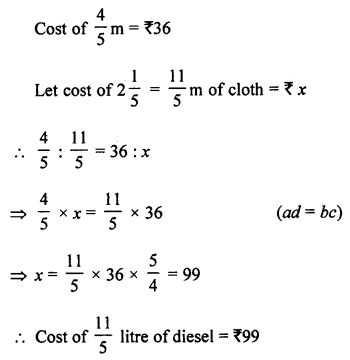## ML Aggarwal Class 7 Solutions for ICSE Maths Chapter 6 Ratio and Proportion Ex 6.3

Question 1.
6 bowls cost ₹ 90. What would be the cost of 10 such bowls?
Solution:
Cost of 6 bowls = ₹ 90
Let cost of 10 bowls = ₹ x
6 : 10 = 90 : x
⇒ 6 × x = 10 × 90 (ad = bc)
x = ₹ 150
Cost of 10 bowls = ₹ 150

Question 2.
Ten pencils cost ₹ 15. How many pencils can be bought with ₹ 72?
Solution:
Cost of 10 pencils = ₹ 15
Let number of pencils will be bought in ₹ 72 = x
10 : x = 15 : 72
x × 15 = 10 × 72 (bc = ad)
x = 48
Number of pencils = 48

Question 3.
400 grams cake costs 80 rupees. How much would a 1.5 kg cake cost?
Solution:
Cost of 400 g cake = ₹ 80
Let cost of 1.5 kg cake = ₹ x
1.5 kg = 1500 gm
400 : 1500 = 80 : x
400 × x – 1500 × 80 (ad = bc)
x = ₹ 300
Cost of 1.5 kg = ₹ 300

Question 4.
A man earns ₹ 18000 in 3 months.
(i) How much time would he take to earn ₹ 30000?
(ii) How much money will he earn in 7 months?
Solution:
A man earns in 3 months = ₹ 18000
Let ₹ 30000 be earned in = x months
18000 : 30000 = 3 : x (ad = bc)
18000 × x = 30000 × 3
x = 5
₹ 30000 will be earn in 5 months
(ii) Let money will be earned in 7 months = ₹ x
3 : 7 = 18000 : x (ad = bc)
3 × x = 7 × 18000
x = 42000
A money of ₹ 42000 will be earned.

Question 5.
12 mangoes weigh 2.4 kg. What is the weight of 8 mangoes?
Solution:
Weight of 12 mangoes = 2.4 kg
Let weight of 8 mangoes = x kg
12 : 8 = 2.4 : x
12 × x = 8 × 2.4
x = 1.6
Weight of 8 mangoes = 1.6 kg

Question 6.
If the weight of 12 sheets of thick paper is 40 grams, how many sheets of the same paper would weigh 2$$\frac { 1 }{ 2 }$$ kilograms?
Solution:
Weight of 12 sheets of paper = 40 grams
Let 2$$\frac { 1 }{ 2 }$$ kg = $$\frac { 5 }{ 2 }$$ × 1000 = 2500 gram,
sheets of thick paper = x sheets
12 : x = 40 : 2500
12 × 2500 = x × 40 (ad = bc)
x = 750
Sheets are 750

Question 7.
A bus consumes 25 litres of diesel in covering a distance of 90 kilometres. How much diesel is needed to cover 288 kilometres?
Solution:
A bus consum 251 of diesel to cover = 90km
Let it will consum x litres of diesel for 288 km
90 : 288 = 25 : x
90 × x = 288 × 25
x = 80
Required diesel = 80 litre

Question 8.
If $$\frac { 4 }{ 5 }$$ metre cloth costs ₹ 36, find the cost of 2$$\frac { 1 }{ 5 }$$ metres of cloth.
Solution:Question 9.
If 15 men can pack 540 parcels per day, how many men are needed to pack 396 parcels per day?
Solution:
15 men can pack = 540 parcels per day
Let 396 parcles be packed by x men
540 : 396 = 15 : x
540 × x = 396 × 5 (ad = bc)
x = 11
Required men = 11

Question 10.
Which is a better buy 12 kg potatoes for ₹ 132 or 16 kg potatoes for ₹ 168?
Solution:
12 kg potatoes for ₹ 132
Cost of 1 kg = $$\frac { 132 }{ 12 }$$ = ₹ 11
and cost of 16 kg = ₹ 168
Cost of 1 kg = $$\frac { 168 }{ 16 }$$ = ₹ 10.50
Second deal is better.
i.e., 16 kg of potatoes for ₹ 168 is better.